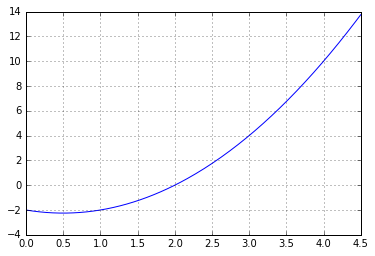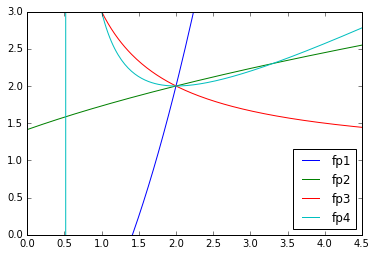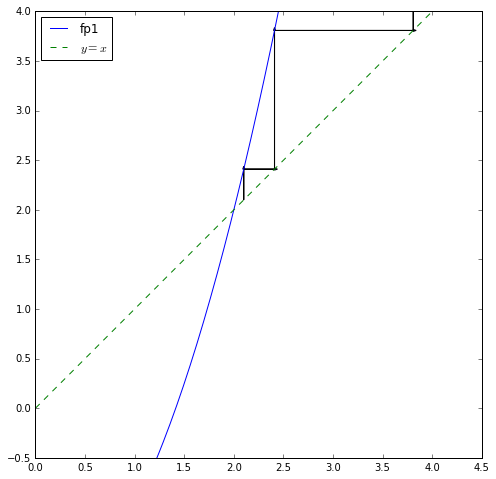# Fixed Point Iteration¶

In :
import numpy as np
import matplotlib.pyplot as pt


Task: Find a root of the function below by fixed point iteration.

In :
x = np.linspace(0, 4.5, 200)

def f(x):
return x**2 - x - 2

pt.plot(x, f(x))
pt.grid()Actual roots: $2$ and $-1$. Here: focusing on $x=2$.

We can choose a wide variety of functions that have a fixed point at the root $x=2$:

(These are chosen knowing the root. But here we are only out to study the behavior of fixed point iteration, not the finding of fixed point functions--so that is OK.)

In :
def fp1(x): return x**2-2
def fp2(x): return np.sqrt(x+2)
def fp3(x): return 1+2/x
def fp4(x): return (x**2+2)/(2*x-1)

fixed_point_functions = [fp1, fp2, fp3, fp4]

In :
for fp in fixed_point_functions:
pt.plot(x, fp(x), label=fp.__name__)
pt.ylim([0, 3])
pt.legend(loc="best")

/usr/local/lib/python3.5/dist-packages/ipykernel/__main__.py:3: RuntimeWarning: divide by zero encountered in true_divide
app.launch_new_instance()

Out:
<matplotlib.legend.Legend at 0x7fd83468d6a0>Common feature?

In :
for fp in fixed_point_functions:
print(fp(2))

# All functions have 2 as a fixed point.

2
2.0
2.0
2.0

In :
z = 2.1; fp = fp1
#z = 1; fp = fp2
#z = 1; fp = fp3
#z = 1; fp = fp4

n_iterations = 4

pt.figure(figsize=(8,8))
pt.plot(x, fp(x), label=fp.__name__)
pt.plot(x, x, "--", label="$y=x$")
pt.gca().set_aspect("equal")
pt.ylim([-0.5, 4])
pt.legend(loc="best")

for i in range(n_iterations):
z_new = fp(z)

pt.arrow(z, z, 0, z_new-z)
pt.arrow(z, z_new, z_new-z, 0)

z = z_new
print(z)

2.41
3.8081000000000005
12.501625610000003
154.29064289260796